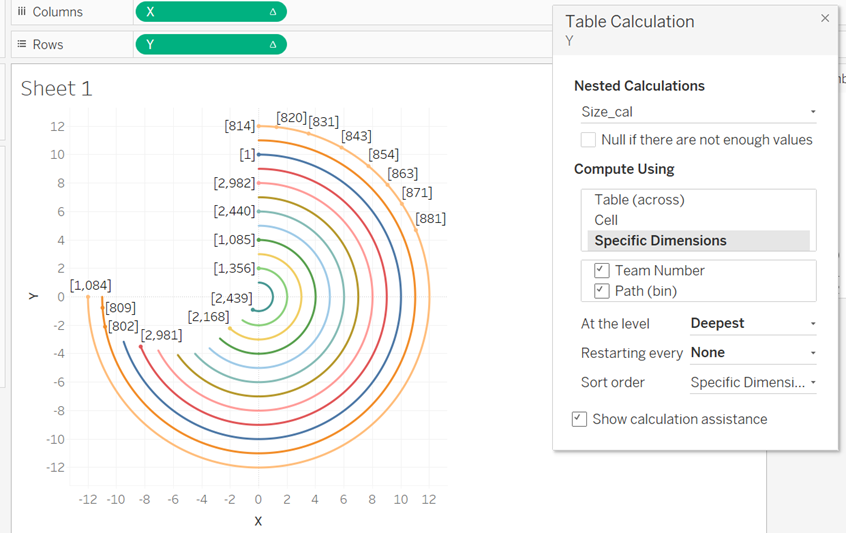top of pageSearch

Radial chart is also known as Polar line chart is useful for displaying cyclical data of several series in a circular form. In a radial visualization , each bar appears in a circle with loger bars that represent larger values.

Let us see how to create amazing radial chart .

Here I am taking a simple dataset contains teamwise result of a competitionCreate a union with same table sheet1. We can see a new column ‘Table Name’ at the endCreate a calculated field Path for starting point 0 and endpoint 270.Create bins for Path with size 1Create an Index calculated field. I used INDEX()-1 because first row of index starts from 1 but Path value starts from 0 to 270RADIANS function converts number from degrees to radians value. SIN funciton to return Sin value and COS function returns Cos value of a number.

Put X on rows and Y on Columns. Put Path(bins) to Columns and make sure that all Missing values are checked.Make Marks to line and move Path(bin) to pathClick on X – Compute Using – Path(bin)

Click on Y – Compute Using – Path(bin)Now to show case teams put Team number to color. We want each team in different circles. So we need to create calculated fields for that

Total_Cal_GrandTotalRank_calc – Will help to create different circles for different teamsNow we need some changes in X and Y values.

Multiply X and Y values with Rank_calcAgain Click on X and Y and make it to Compute Using Path(bin)Go to Edit table Calculations – Nested Calculations – Rank_calc

Select Team Number instead of Path for both X and YWe have different circles for different teams. Next we need to create a calculate field Total Grand totalWe have to create Percentage calculationCreate a calculated field that helps us to change the size of radials based on the percentage.Make changes in our X and Y calculatiosNo radial in our chart . Inorder to make radial we need to repeat the process

Go X an Y Make it Compute Using Path(bin)Edit table calculations. Again change Rank_calc – Path to Team NumberAnd select Size_cal in our nested calculation - Select both Path(bin) and Team Number and move Team Number to topFor Total_gTotal follow the above steps alsoFollow the same steps for Y calculations as wellWe can see that our radial chart has taken the shape as we neededHave you noticed exercise ring in apple watch?. Its a radial chart in real life scenario